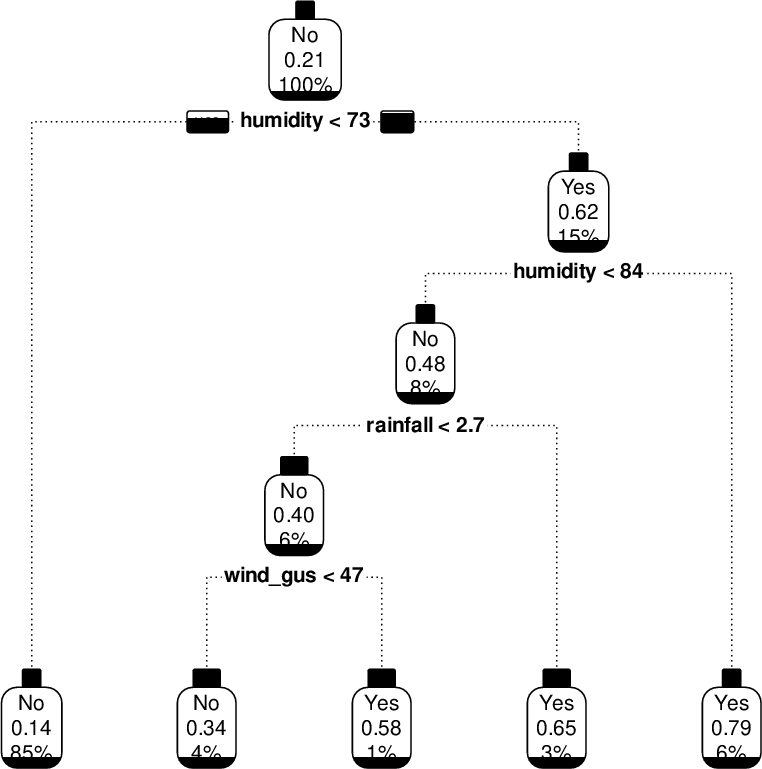Data Science Desktop Survival Guide by Graham WilliamsDesktop Survival Project Home Preface Data Science Introducing R R Constructs R Tasks R Strings R Read, Write, and Create Data Template Data Exploration Data Wrangling Data Visualisation Statistics ML Template ML Scenarios ML Activities ML Applications ML Algorithms Cluster Analysis Decision Trees Computer Vision Graph Data Privacy Literate Data Science Coding with Style Resources Bibliography Index

## Enhanced Plots: Draw Branches as Dotted Linesprp(model, type=2, extra=106, nn=TRUE, fallen.leaves=TRUE,     branch.lty=3) The branch.lty= option allows us to specify the type of line to draw for the branches. A dotted line is attractive as it reduces the dominance of the branches whilst retaining the node connections. Other options are just the standard values for line type in R:
 plot(c(0,1), c(0,0), type="l", axes=FALSE, xlab=NA, ylab=NA, lty=2) plot(c(0,1), c(0,0), type="l", axes=FALSE, xlab=NA, ylab=NA, lty="dashed") plot(c(0,1), c(0,0), type="l", axes=FALSE, xlab=NA, ylab=NA, lty="44")